## Potential Energy in an External Field

### The potential energy of a single charge:

The external electric field E and the corresponding external potential V may vary from point to point. By definition, V at a point P is the work done in bringing a unit positive charge from infinity to the point P.

Work done in bringing a charge q from infinity to the point P in the external field is qV. This work is stored in the form of potential energy of q. If the point P has position vector r relative to some origin,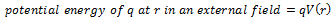### The potential energy of a system of two charges in an external field:

Work done in bringing the charge q1 from infinity to r1 is q1 V(r1). Consider the work done in bringing q2 to r2. In this step, work is done not only against the external field E but also against the field due to q1.

Work done on q2 against the external field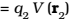Work done on q2 against the field due to q1By superposition principle for fields, add up the work done on q2 against the two fields. Work done in bringing q2 to r2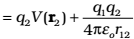Thus, Potential energy of the system = the total work done in assembling the configuration### The potential energy of a dipole in an external field:

Consider a dipole with charges q1 = +q and q2 = -q placed in a uniform electric field E.

In a uniform electric field, the dipole experiences no net force; but experiences a torque τ given by which will tend to rotate it (unless p is parallel or antiparallel to E).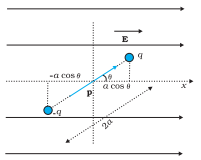Suppose an external torque τext is applied in such a manner that it just neutralizes this torque and rotates it in the plane of paper from angle θ0 to angle θ1 at an infinitesimal angular speed and without angular acceleration. The amount of work done by the external torque will be given by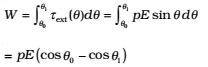This work is stored as the potential energy of the system. We can then associate potential energy U(θ) with an inclination θ of the dipole. Similar to other potential energies, there is a freedom in choosing the angle where the potential energy U is taken to be zero. A natural choice is to take θ0 = π / 2.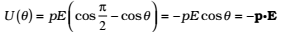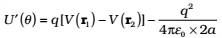Here, r1 and r2 denote the position vectors of +q and –q. Now, the potential difference between positions r1 and r2 equals the work done in bringing a unit positive charge against field from r2 to r1.

The displacement parallel to the force is 2acosθ. Thus,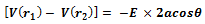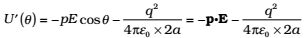We note that U′(θ) differs from U(θ ) by a quantity which is just a constant for a given dipole. Since a constant is insignificant for potential energy.

We can now understand why we took θ0 = π/2. In this case, the work done against the external field E in bringing +q and – q are equal and opposite and cancel out, i.e., q [V (r1) – V (r2)] = 0.